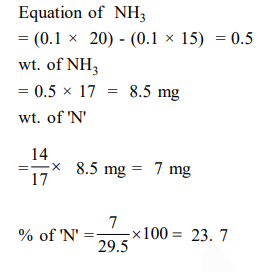# Solve this following`
Question:

$29.5 \mathrm{mg}$ of an organic compound containing nitrogen was digested according to Kjeldahl's method and the evolved ammonia was absorbed in $20 \mathrm{~mL}$ of $0.1 \mathrm{M} \mathrm{HCl}$ solution. The excess of the acid required 15 $\mathrm{mL}$ of $0.1 \mathrm{M} \mathrm{NaOH}$ solution for complete neutralization. The percentage of nitrogen in the compound is

1. $29.5$

2. $59.0$

3. $47.4$

4. $23.7$

Correct Option: , 4

Solution: